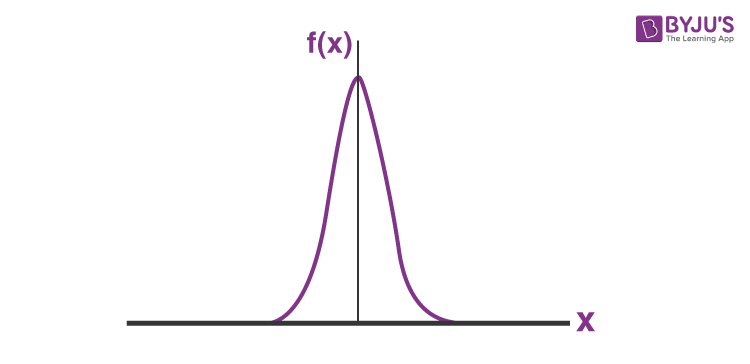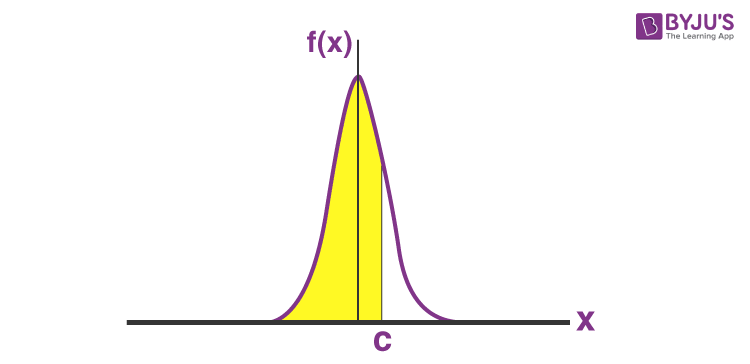# How to Find Probability of Normal Distribution

A type of continuous probability distribution for a real-valued random variable is a normal distribution. It is also called Gaussian or Gauss or Laplace–Gauss distribution. Normal distributions are important in statistics. They are used in the natural and social sciences to represent real-valued random variables whose distributions are not known. The normal distribution is a probability function that explains how the values of a variable are distributed. A random variable with a Gaussian distribution is normally distributed and is called a normal deviate. In this article, we will learn how to find the probability of normal distribution.

## What is Normal Distribution?

Normal distribution is a probability function that explains how the values of a variable are distributed. It is defined by the probability density function for a continuous random variable in a system. This type of statistical data distribution pattern occurs in phenomena, such as blood pressure, height, etc.

## How to Calculate Probability of Normal Distribution?

Normal distribution is a continuous distribution having the following algebraic expression for the probability density.

$f(x) = \frac{1}{\sigma \sqrt{2\pi }}e^{\frac{-1}{2}(\frac{x-\mu }{\sigma })^{2}}$

Here μ is the mean of the distribution and σ is the standard deviation. The general form of the normal distribution is given below. The distribution is symmetric about the mean (peak). Since this distribution is continuous, integral calculus is needed to directly calculate associated probabilities.The probability P(X ≤ x) can be given as

$P(X\leq c)= \int_{-\infty }^{c}f(x)dx = \frac{1}{\sigma \sqrt{2\pi }}\int_{-\infty }^{c}e^{\frac{-1}{2}(\frac{x-\mu }{\sigma })^{2}}dx$

This calculates the area under the curve from the extreme left (negative infinity) to x = c, refers to the shaded region shown below.We can find the probabilities of the form P(a < X ≤ b) in such cases, the shaded region would be more limited. Remember that the probability of a distribution is associated with the area under the curve for a particular range of values. The area under the entire normal curve that extends to positive and negative infinity is unity.

Test your Knowledge on Normal distribution